### IMO Shortlist 1984 problem 15

Kvaliteta:
Avg: 0,0
Težina:
Avg: 0,0
Angles of a given triangle$ABC$ are all smaller than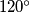$120^\circ$. Equilateral triangles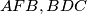$AFB, BDC$ and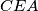$CEA$ are constructed in the exterior of$ABC$.

(a) Prove that the lines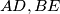$AD, BE$, and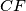$CF$ pass through one point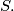$S.$

(b) Prove that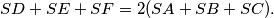$SD + SE + SF = 2(SA + SB + SC).$
Izvor: Međunarodna matematička olimpijada, shortlist 1984